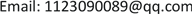﻿ 点染色和边赋权 Vertex-Colors and Edge-Weights

Vol. 08  No. 04 ( 2019 ), Article ID: 29802 , 5 pages
10.12677/AAM.2019.84074

Vertex-Colors and Edge-Weights

Huijuan Zhang

Zhejiang Normal University, Jinhua ZhejiangReceived: Mar. 31st, 2019; accepted: Apr. 15th, 2019; published: Apr. 22nd, 2019ABSTRACT

Lyngsie, Thomassen and Zhong  on the base of 1-2-3-conjection proposed a conjecture that strengthens the four-color theorem: Every graph $G$ with no isolated edges has a mapping $w:E\left(G\right)\to \left\{1,2,3\right\}$ , so that for any two adjacent vertices $u\text{and}v$ , $\underset{e\in E\left(u\right)}{\sum }w\left(e\right)\ne \underset{e\in E\left(v\right)}{\sum }w\left(e\right)\left(mod4\right).$ We say satisfy the above conditions edge weighting $w$ is 3-edge weighting 4-coloring of a graph $G$ . This conjecture is considerably stronger than the four-color theorem. In this paper, we prove that Lyngsie, Thomassen and Zhong conjecture holds for trees. By using the four-color theorem, we prove that every planar graph has a 4-edge weighting 4-coloring.

Keywords:Tree, Planer Graph, Connected Graph, Edge Weighting Vertex ColoringLyngsie，Thomassen和Zhong  在1-2-3-猜想的基础上提出了一个强化4-色定理的猜想：对于任意不含孤立边的平面图 $G$，存在 $G$ 的一个边赋权 $w:E\left(G\right)\to \left\{1,2,3\right\}$，使得对任意相邻的两个顶点 $u,v$，有 $\underset{e\in E\left(u\right)}{\sum }w\left(e\right)\ne \underset{e\in E\left(v\right)}{\sum }w\left(e\right)\left(mod4\right).$ 我们称满足上述条件的边赋权 $w$$G$ 的一个3-边赋权4-染色。这是一个比4-色定理强很多的猜想。在本文中我们证明了阶数至少为3的树满足这个猜想。另外，利用4-色定理，我们证明了每一个平面图 $G$ 存在一个4-边赋权4-染色。1. 引言

Karoński，Luczak和Thomason证明了没有孤立边的3-可染的连通图满足1-2-3-猜想。2010年 Kalkowski，Karoński和Pfender  证明了没有孤立边的连通图，存在一个5-边赋权染色。2017年，Wu，Zhang和Zhu  等人证明了4-可染的4-边连通图存在3-边赋权染色。Lyngsie，Thomassen和Zhong  提出了强化4-色定理的猜想。Lyngsie等人证明了4-可染的4-边连通图和三角化的平面图满足这个猜想。

2. 定理1的证明

$L\left(v\right)=\left\{1,2,3\right\}$，如果 $v$$T$ 的叶子点；

$L\left(v\right)=\left\{0,1,2,3\right\}$，否则。

$\underset{e\in E\left(v\right)}{\sum }w\left(e\right)\equiv c\left(v\right)\left(\mathrm{mod}4\right)$

$c$$T$ 的一个好的点染色， $c\left(v\right)\in L\left(v\right)$

Case 1： $d\left({u}^{*}\right)=2$

${T}^{\prime }=T-u$，由归纳假设对任意的点 $v\in V\left({T}^{\prime }\right)$ 存在一个边赋权 ${w}^{\prime }:E\left({T}^{\prime }\right)\to \left\{1,2,3\right\}$$\underset{e\in E\left(v\right)}{\sum }{w}^{\prime }\left(e\right)\equiv {c}^{\prime }\left(v\right)\left(\mathrm{mod}4\right)$

$\underset{e\in E\left(v\right)}{\sum }w\left(e\right)\equiv c\left(v\right)\left(\mathrm{mod}4\right)$

$c$$T$ 的一个好的染色， $c\left(v\right)\in L\left(v\right)$

Case 2： $d\left({u}^{*}\right)\ge 3$

$\underset{e\in E\left(v\right)}{\sum }{w}^{\prime }\left(e\right)\equiv {c}^{\prime }\left(v\right)\left(\mathrm{mod}4\right)$

$\underset{e\in E\left(v\right)}{\sum }w\left(e\right)\equiv c\left(v\right)\left(\mathrm{mod}4\right)$

3. 定理2的证明

Case 1：如果图 $G$ 是二部图。

Case 2：如果图 $G$ 是非二部图。

$c:V\left(G\right)\to \left\{0,1,2,3\right\}=\left\{{g}_{1},{g}_{2},{g}_{3},{g}_{4}\right\}$ 是一个好的点染色。通过置换颜色类，我们可以假设顶点颜色的总和是偶数，假设 $\underset{v\in V\left(G\right)}{\sum }c\left(v\right)=2h$。令其中一条边的权重为 $h\left(\mathrm{mod}4\right)$ 和剩余其他边的权重为0，所以顶点权重的总和是 $2h$${n}_{1}{g}_{1}+{n}_{2}{g}_{2}+{n}_{3}{g}_{3}+{n}_{4}{g}_{4}=2h$。我们现在尝试修改权重，使得顶点的权重总和不变，直到所有染颜色 $i$ 的顶点有权重 ${g}_{i},1\le i\le 4$。假设存在一个染颜色 $i$ 的顶点 $u$ 有错误的权重 $g\ne {g}_{i}$ ；因为 ${n}_{1}{g}_{1}+{n}_{2}{g}_{2}+{n}_{3}{g}_{3}+{n}_{4}{g}_{4}=2h$ 那么存在另一个顶点 $v\in V\left(G\right)$ 的权重也是错误的，选择一个从 $u$$v$ 的偶长途径，这总是可以存在的，除非图 $G$ 是二部图。遍历这个途径，给途径上的边交替增加 ${g}_{i}-g,g-{g}_{i},{g}_{i}-g,g-{g}_{i},\cdots$。这个操作保持点的权重的总和，除了点 $u$ 和点 $v$ 其他所有点的权重不变，并且生成了一个正确的点。因此，重复的应用以上操作给出所需的权重。定理证毕。

Vertex-Colors and Edge-Weights[J]. 应用数学进展, 2019, 08(04): 664-668. https://doi.org/10.12677/AAM.2019.84074

1. 1. Lyngsie, K.S., Thomassen, C. and Zhong, L. (2018) Four Vertex-Colors and Three Edge-Weights. AMS Subject Clas-sifications, 05C10, 05C15, 05C22.

2. 2. Karoński, M., Luczak, T. and Thomason, A. (2004) Edge Weights and Vertex Colours. Journal of Combinatorial Theory, Series B, 91, 151-157. https://doi.org/10.1016/j.jctb.2003.12.001

3. 3. Kalkowski, M., Karon’ski, M. and Pfender, F. (2010) Ver-tex-Coloring Edge-Weightings: Towards the 1-2-3-Conjecture. Journal of Combinatorial Theory, Series B, 100, 347-349. https://doi.org/10.1016/j.jctb.2009.06.002

4. 4. Wu, Y.Z., Zhang, C.Q. and Zhu, B.X. (2017) Vertex-Coloring 3-Edge-Weighting of Some Graphs. Discrete Mathematics, 340, 154-159. https://doi.org/10.1016/j.disc.2016.08.011